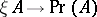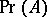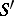# Gödel completeness theorem

The following statement on the completeness of classical predicate calculus: Any predicate formula that is true in all models is deducible (by formal rules of classical predicate calculus). The theorem proves that the set of deducible formulas of this calculus is, in a certain sense, maximal: it contains all purely-logical laws of set-theoretic mathematics. K. Gödel's proof  yields a means of constructing a countermodel (i.e. model for the negation) of any formulathat is not-deducible in the Gentzen formal system without cut-rule. There are also proofs based on extensions of the system of formulas to a maximal system, and also proofs involving the use of algebraic methods. The theorem together with the proof has been generalized to include calculi with equality. Another direction is a generalization to arbitrary sets of formulas: Any consistent set of formulas has a model (a setis called consistent if for anyit is not possible to deduce). Gödel's proof yields a model, with terms as its elements, for a consistent set of formulas. Such models constitute a starting point in many meta-mathematical studies on set theory. Another application of models of terms is the Löwenheim–Skolem theorem: If a denumerable set of formulas has a model, then it has a denumerable model. Gödel's proof itself can be carried out in set theory without the axiom of infinity, i.e. by arithmetical means. One obtains thus a constructive form of Gödel's completeness theorem (Bernays' lemma): For each predicate formulait is possible to find a substitutionof arithmetical predicates for predicate variables such thatis deducible in formal arithmetic. Hereis the arithmetical formula that says thatis deducible. Thus, forto be deducible it is sufficient for it to be true in the model defined by the substitution. Bernays' lemma is used to construct models of a formal systemin a systemif inthe consistency ofhas been proved.